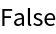#Function Repository Resource:

# CausalInvariantQ

Determine whether a given multiway system is causal invariant

Contributed by: Jonathan Gorard
 ResourceFunction["CausalInvariantQ"][rules,init,n] returns True if the multiway system with the specified rules is causal invariant after n steps, starting with initial conditions init, and False otherwise. ResourceFunction["CausalInvariantQ"][rules→sel,init,n] uses the function sel to select which of the events obtained at each step to include in the evolution.

## Details and Options

Rules can be specified in the following ways:
 {"lhs1"->"rhs1",…} string substitution system {{l11,l12,…}->{r11,r12,..},…} list substitution system [ rules ] string or list substitution system [ rules ] cellular automaton system "type"→rules system of the specified type assoc system with properties defined by assoc
Supported rule types include:
 "StringSubstitutionSystem" rules given as replacements on strings "ListSubstitutionSystem" rules given as replacements on lists "CellularAutomaton" rules given as a list of CellularAutomaton rule specifications "WolframModel" rules given as replacements on hypergraphs
When rules are specified by an explicit association, the following elements can be included:
 "StateEvolutionFunction" gives the list of successors to a given state "StateEquivalenceFunction" determines whether two states should be considered equivalent "StateEventFunction" gives the list of events applicable to a given state "EventApplicationFunction" applies an event to a given state "EventDecompositionFunction" decomposes an event into creator and destroyer events for individual elements "SystemType" system type name "EventSelectionFunction" determines which events should be applied to a given state
The event selection function sel in ResourceFunction["CausalInvariantQ"][rulessel,] can have the following special forms:
 "Sequential" applies the first possible replacement (sequential substitution system) "Random" applies a random replacement {"Random",n} applies n randomly chosen replacements "MaxScan" applies the maximal set of spatially-separated replacements (strings only)
The initial condition for ResourceFunction["CausalInvariantQ"] is a list of states appropriate for the type of system used.
ResourceFunction["CausalInvariantQ"][rules,"string",] is interpreted as ResourceFunction["CausalInvariantQ"][rules,{"string"},].
ResourceFunction["CausalInvariantQ"] accepts both individual rules and lists of rules, and likewise for initial conditions.
Options for ResourceFunction["CausalInvariantQ"] include:
 "IncludeStepNumber" whether to label states and events with their respective step numbers "IncludeStateID" whether to label states and events with unique IDs
Causal invariance is a property of multiway systems in which all branches of evolution history yield causal networks that are isomorphic as acyclic graphs.
In the case of an abstract rewrite system it is a sufficient condition for confluence (or the Church-Rosser property), and in the case of a WolframModel it is equivalent to Lorentz covariance.

## Examples

### Basic Examples (4)

Determine causal invariance for two string substitution systems:

 In:=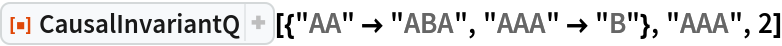Out=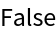In:=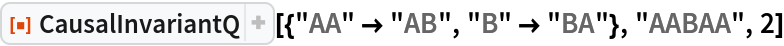Out=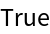Different event selection functions can lead to different causal invariance behavior:

 In:=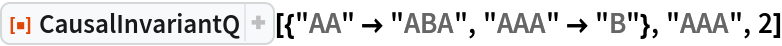Out=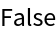In:=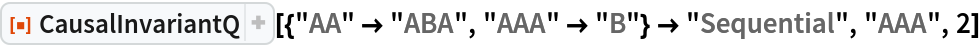Out=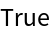CausalInvariantQ can handle Wolfram Models and other system types:

 In:=Out=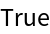In:=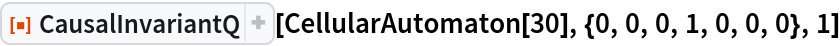Out=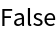In:=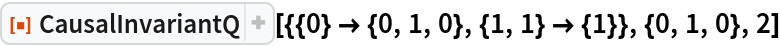Out=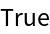Preventing identical states from being merged, by including step numbers and/or state IDs, can break causal invariance:

 In:=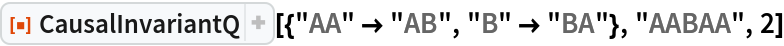Out=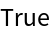In:=Out=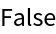### Scope (10)

#### System Types (5)

CausalInvariantQ supports both string and list substitution systems:

 In:=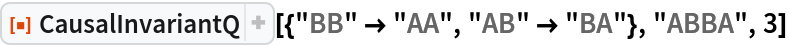Out=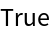In:=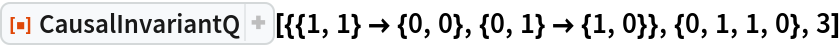Out=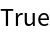Lists can contain arbitrary symbolic elements:

 In:=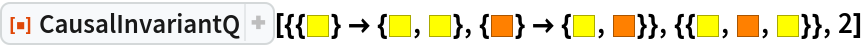Out=Give an explicit substitution system rule:

 In:=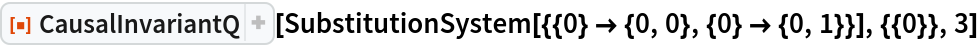Out=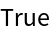An alternative method of specifying that a substitution system should be used:

 In:=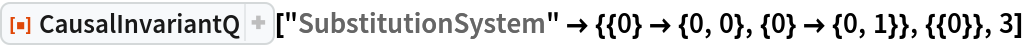Out=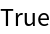CausalInvariantQ also supports multiway generalizations of cellular automata:

 In:=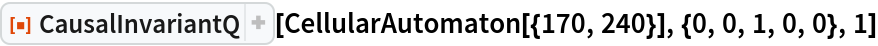Out=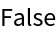In:=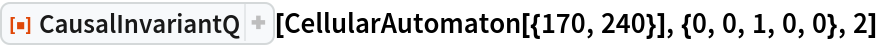Out=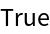CausalInvariantQ also supports multiway generalizations of Wolfram Models:

 In:=Out=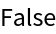In:=Out=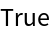Construct a multiway evolution by explicitly specifying an association:

 In:=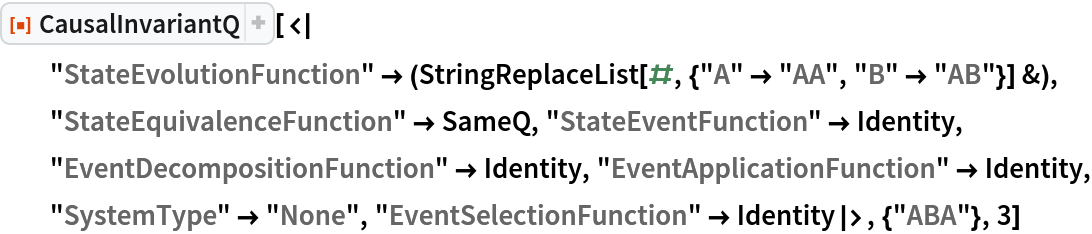Out=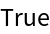#### Rules and Initial Conditions (2)

CausalInvariantQ accepts both individual rules and lists of rules:

 In:=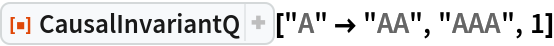Out=In:=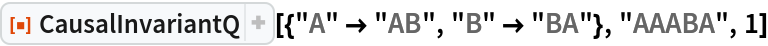Out=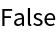Likewise for initial conditions:

 In:=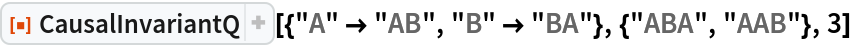Out=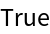#### Event Selection Functions (3)

Apply only the first possible event at each step:

 In:=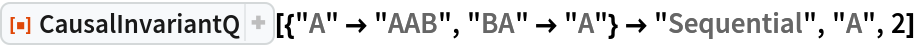Out=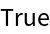Apply the first and last possible events at each step:

 In:=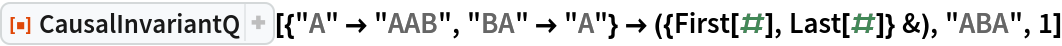Out=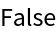Use a greedy-style algorithm to apply the maximal set of non-conflicting events at each step (strings only):

 In:=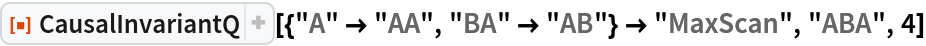Out=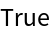### Options (3)

Explicitly specify the type of rule:

 In:=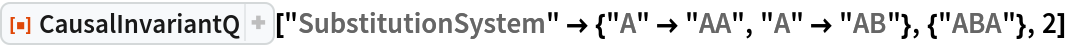Out=In:=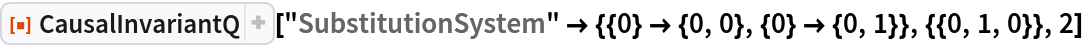Out=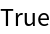#### Step Numbers and State IDs (3)

By default, equivalent states are merged across all time steps:

 In:=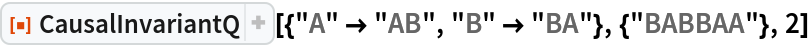Out=Merging of equivalent states across different time steps can be prevented by including step numbers:

 In:=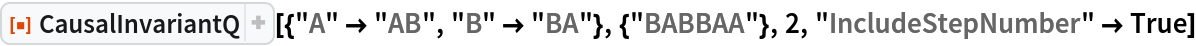Out=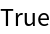Merging of equivalent states at the same time step can be prevented by also including state IDs:

 In:=Out=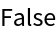### Applications (2)

#### Causal Invariant Rules (2)

Prove that the Xor and And functions are causal invariant (due to associativity):

 In:=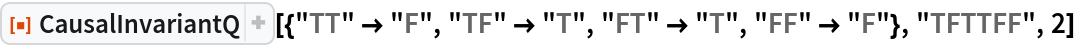Out=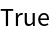In:=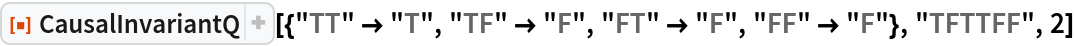Out=On the other hand, the Nand function is not associative, so it is not causal invariant:

 In:=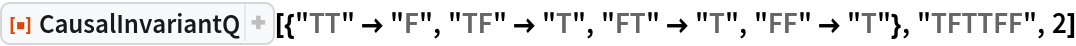Out=